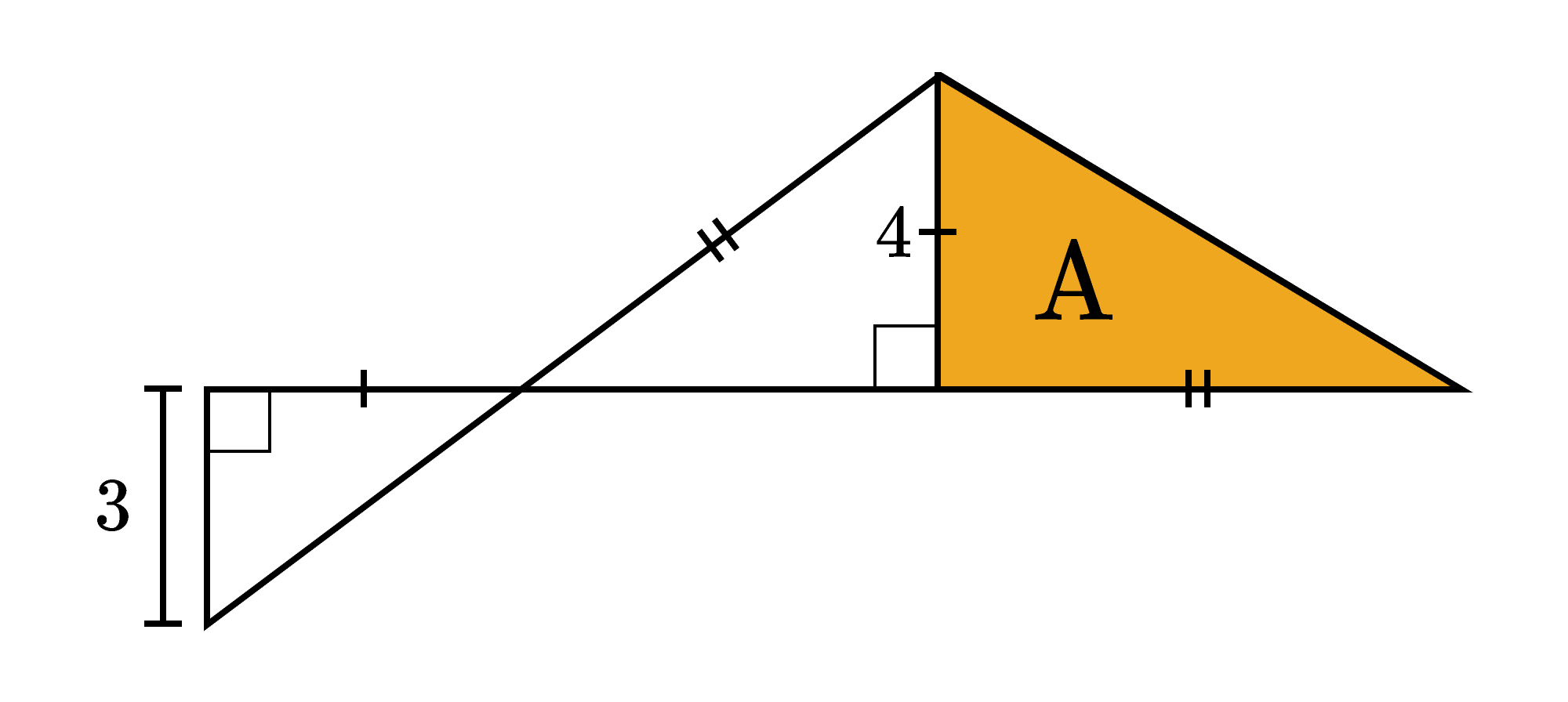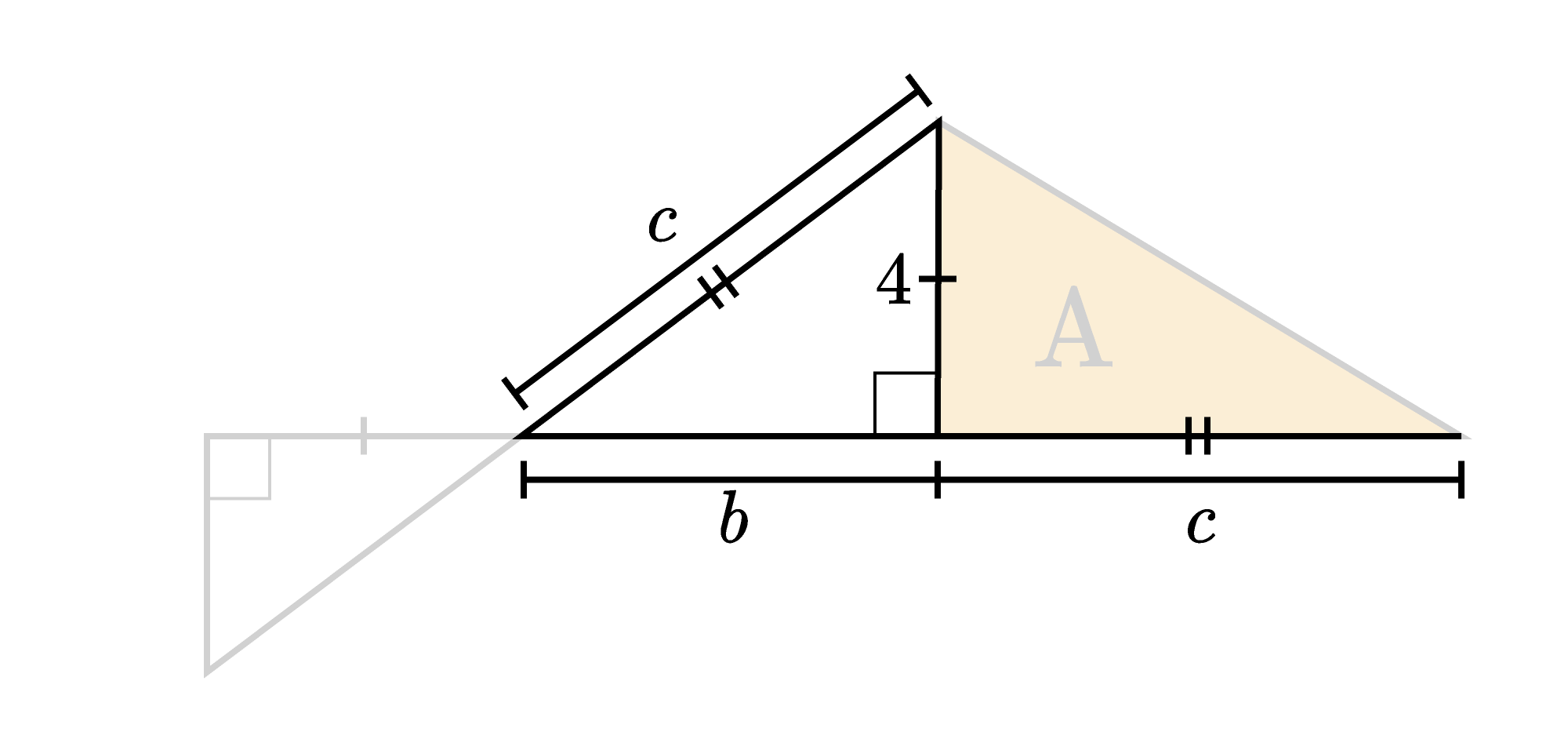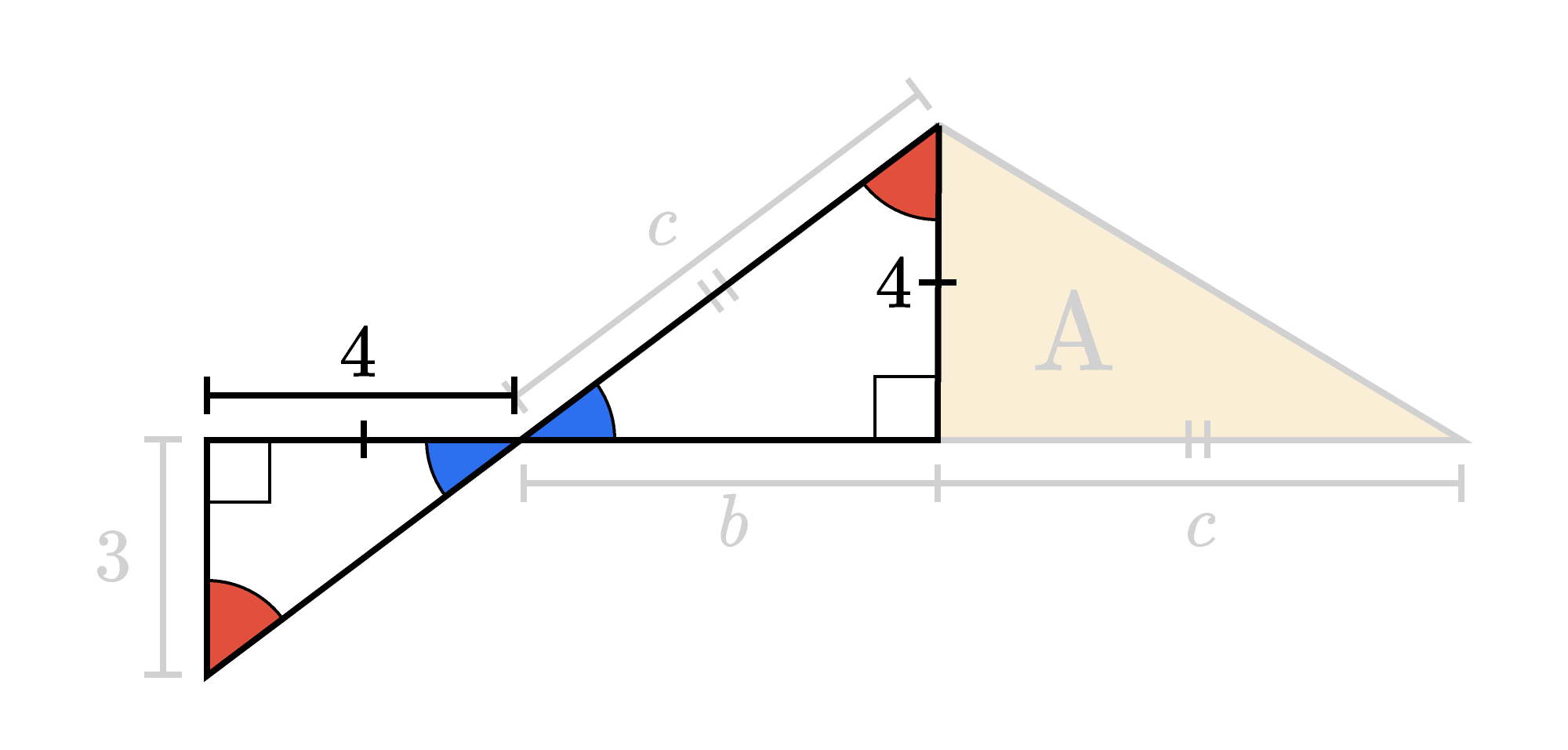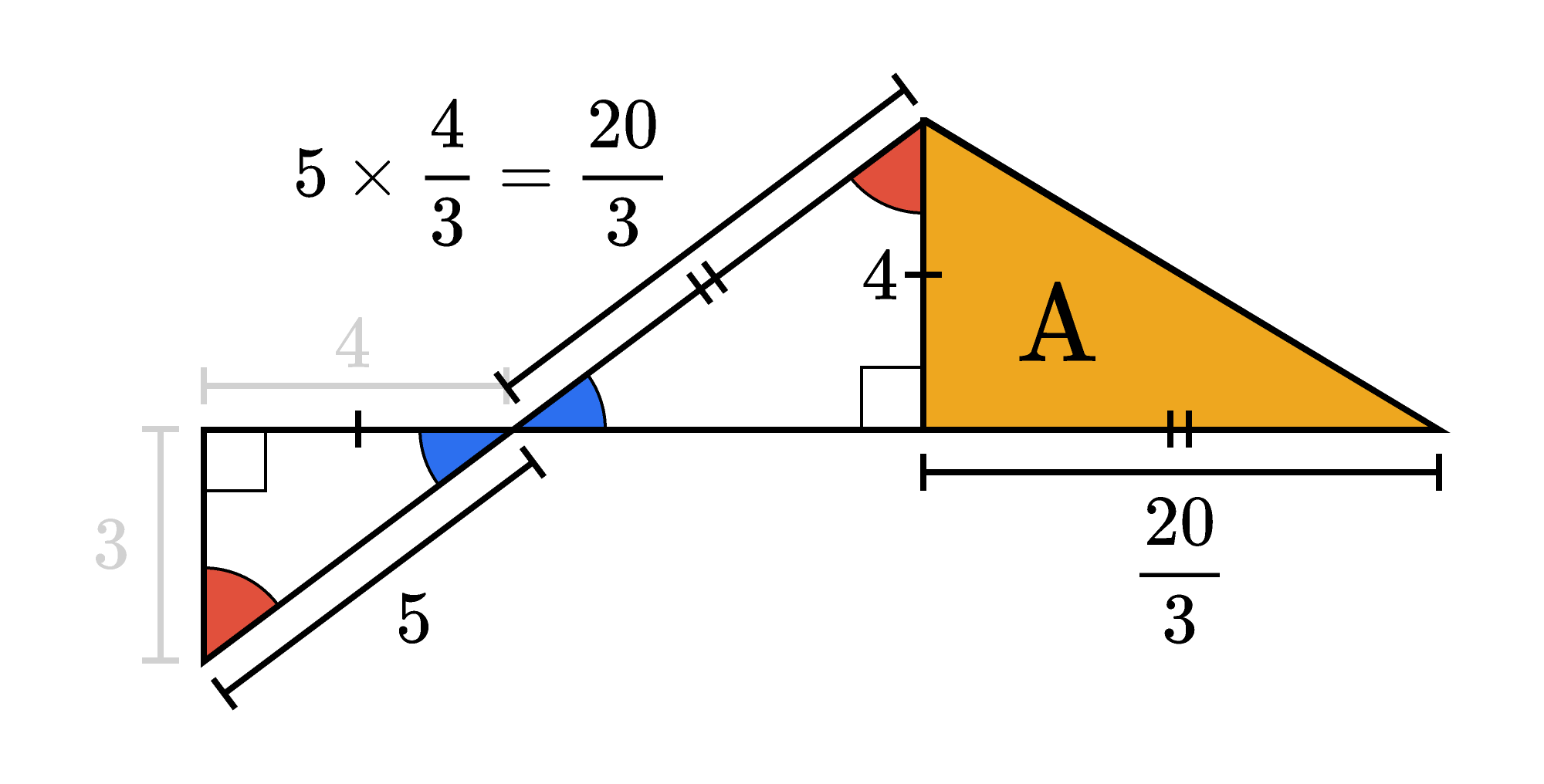Back

## Diagonal Thinking

How can we find $A,$ the area of the colored triangle, when we're only told two lengths in the figure?Keep reading to see one way we can do this, or skip straight to today's challenge if you feel ready for a tougher problem.

When first starting a problem, the wishful thinking strategy can help to point you in the right direction. When using it on this problem, you might think as follows:

I know the height of the colored triangle. If I only knew the length of the base, then I could find the area with the formula $A = \frac12 bh.$

The base of the colored triangle has a pair of tick marks, and so does the hypotenuse of the middle triangle. This means the two segments have the same length or are congruent. So, now, you might think as follows:

I know the length of one leg of the middle triangle. If I only knew the length of the other leg, then I could use the Pythagorean theorem to find the length of the hypotenuse. Then I would have the missing leg of the colored triangle.Let's see if there is anything in the diagram we haven't thought about yet. One leg of the left triangle has a single tick mark, so it's congruent to the known leg of the middle triangle. We now know both leg lengths of the left triangle; maybe we can somehow use that to help to find the missing leg of the middle triangle.

Two angles of the left and middle triangles are formed by a pair of crossing lines. This means those angles are congruent. They also each have a right angle, so the triangles have two pairs of congruent angles. Then, for either triangle, all three angle measures add up to $180^\circ,$ so the other pair of angles must also be congruent.A pair of triangles with three pairs of congruent angles are similar. We know the ratio of the short leg lengths is $\frac43,$ so we could multiply that ratio by $4$ to get the missing leg of the middle triangle: $4 \times \frac43 = \frac{16}{3}.$

We found that leg of the middle triangle, so we could use the Pythagorean theorem to find the hypotenuse:

\begin{aligned} a^2 + b^2 & = c^2 \\ 4^2 + \left(\frac{16}{3}\right)^2 & = c^2 \\ &\ \ \vdots \end{aligned}

Sometimes things get messy, and it might help to change course. The left triangle legs don't have fractional lengths. Why don't we use the Pythagorean theorem to find its hypotenuse instead, and then scale that length up to the middle triangle's hypotenuse?

\begin{aligned} 3^2 + 4^2 & = c^2 \\ 9 + 16 & = c^2 \\ 25 & = c^2 \\ 5 & = c \end{aligned}

Scaling this length up, the middle triangle's hypotenuse has length $5 \times \frac43 = \frac{20}{3}.$ The colored triangle's missing leg has the same length, so we have all the information we need to answer the question.The area of the colored triangle is

\begin{aligned} A & = \frac12 bh \\ & = \frac12 \times \frac{20}{3} \times 4 \\ & = 2 \times \frac{20}{3} \\ & = \frac{40}{3}, \\ \end{aligned}

or $13 \frac13 = 13.\overline{3}.$

The problem below is different, but think about how you can use similar tools to solve it. Pay attention to your steps; it might be helpful to adjust course at some point as we did here.

# Today's Challenge

What is the length of this isosceles trapezoid's diagonal?×

Problem Loading...

Note Loading...

Set Loading...